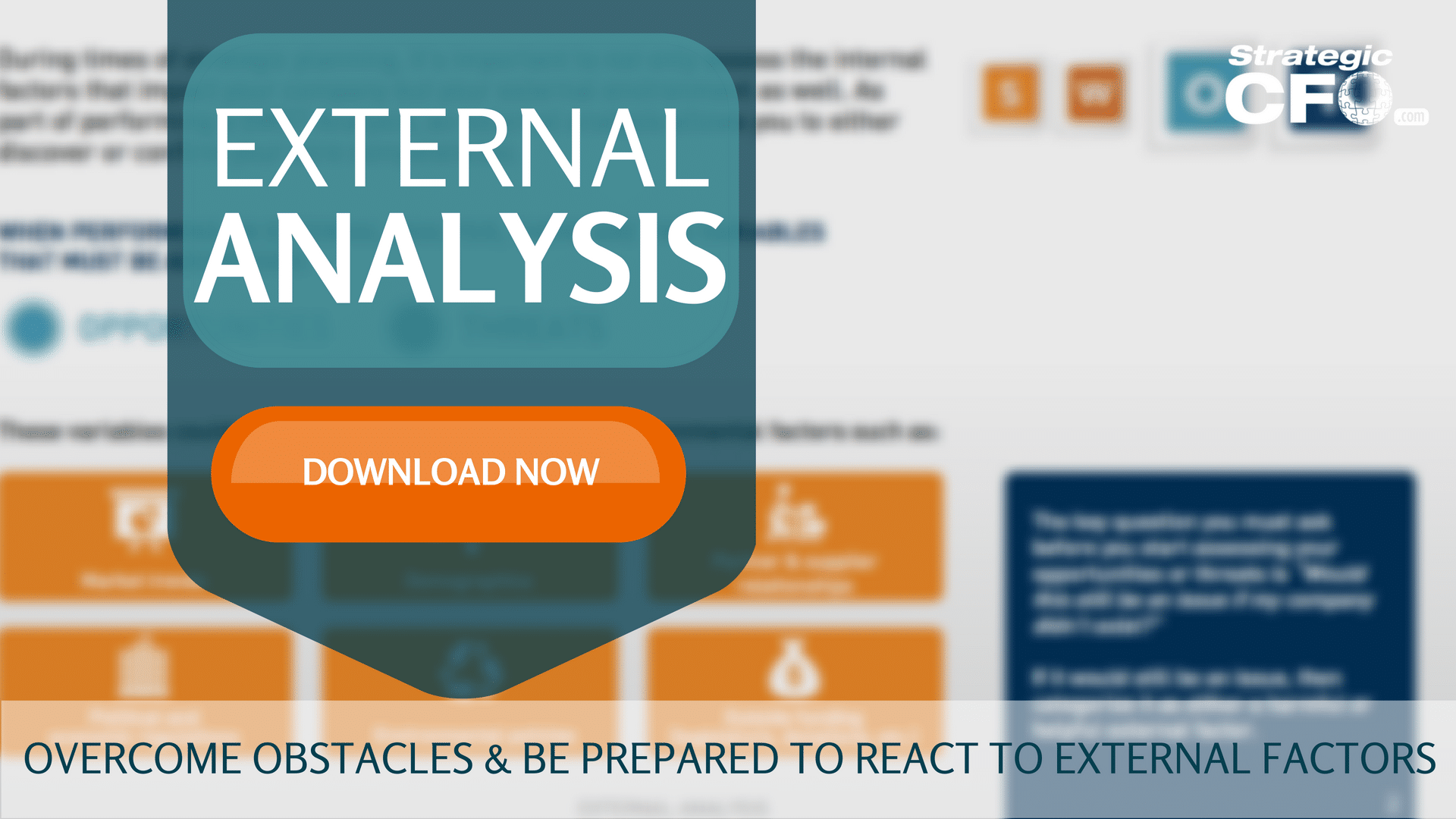# Interest Rate Risk Definition

Interest rate risk is the risk or volatility associated with bonds or long term debt as their interest rates, coupon, yield to maturity, and maturity dates move within the market.

## Factors of Interest Rate Risk

There are typically five types of interest rate risk on bonds and debt instruments as follows:

1) Bond prices and their yields are inversely related. Thus, if a interest rate increases the bond price falls or drops to a discount, and if the interest rate drops the bond prices rises or is considered at a premium. The fluctuations in the market is an interest rate risk that must be accounted for accordingly when investing.

2) The longer the maturity the more sensitive a bond or debt instrument is to interest rate changes. As a bond comes closer to its maturity the price fluctuates less and less from changes in the market. This means that a shorter term security has less interest rate risk.

3) An increase in interest rates will yield a much larger change in a bond than a decrease of the same amount. This means that a bond has the ability to lose its overall value in price than it does in gaining or selling at a premium.

4) Prices of low coupon bonds are much more sensitive to market yield changes than the prices of higher coupon bonds.

5) A bond or debt instrument’s price is much more sensitive if that particular bond has a lower yield to maturity. Thus, the higher the yield to maturity the less sensitive the bond price.

Note: None of these factors matter if a person plans on holding a bond or debt instrument until its maturity. If a person holds a bond until its maturity the fact that interest rates fluctuate is irrelevant because all bonds pay coupons and finally the face value at maturity. This means that this person will automatically make the desired return and therefore need not worry about interest rate risk measures.

### Interest Rate Risk Example

Chuck wants to invest in a debt instrument, and comes across some lucrative bonds. He has narrowed the search down to two, and is trying to decide between bond A and bond B. Both bonds pay a coupon of 8% and have a current yield to maturity of 6%. The only large difference between them is that the maturity for bond A is 5 years and B has a maturity of 30 years. After consulting with a close friend Chuck decides to buy bond A because his friend tells him there is less interest rate risk inherent in bond A.

Download your free External Analysis whitepaper that guides you through overcoming obstacles and preparing how your company is going to react to external factors.Strategic CFO Lab Member Extra

Access your Projections Execution Plan in SCFO Lab. The step-by-step plan to get ahead of your cash flow.